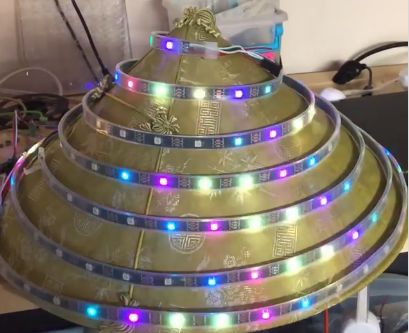# Mapping a cone?

Now I have a conical hat I’d like to map. Its 8 inches high, and 16 inches wide, making it a 45 degree angle from base to tip. I get exactly 7 wraps out a 5m roll of 150 pixels total.

I would love some help mapping this, thanks!Thats a bit harder. Its tempting to do something like Mapping a Helix except the radius shrinks or grows with the for loop’s `i` variable, but the pixel distance gets messed up.

I turned to a bit of googling and calculus. Found the formula for a conical helix, and then integrating to find the arc distance to space out pixels. I don’t have time to dig further to figure out how to find the radius and spacing to match a specific number of pixels given 7 loops, but it’s easy to play with the numbers until things get really close.

``````function (pixelCount) {
loops = 7
r = 7.3
pixelSpacing = .5
resolution = .000005 //controls the resolution of the integral
a = Math.PI * 2 * loops
map = []

function conicalHelix(t, r, a) {
z = t
x = (1-t) * r * Math.cos(t * a)
y = (1-t) * r * Math.sin(t * a)
return [x,y,z]
}

function conicalHelixArcLength(t1, t2, r, a) {
h1 = conicalHelix(t1, r, a)
h2 = conicalHelix(t2, r, a)
dh = [h1 - h2, h1 - h2, h1 - h2]
return Math.sqrt(dh*dh + dh*dh + dh*dh)
}

for (t = 0, i = 0; i < pixelCount; i++) {
//integrate arc lengths until we cover the distance to our next pixel
l = 0;
while (l < pixelSpacing) {
l += conicalHelixArcLength(t, t+resolution, r, a)
t += resolution
}
map.push(conicalHelix(t, r, a))
}
return map
}
``````

2 Likes

oh wow thanks a lot! Ill post progress!

Trying to get this to work, I do know on the strip I have that the distance between pixels is 1.31 inches (since its 30/m)

Im curious about the 7.3 number for ‘r’. was that just something that was adjusted from 8 since the diameter is 16?

It’s all relative. I experimented with the numbers until I had about 7 loops.